# Dyed Nylon Yarn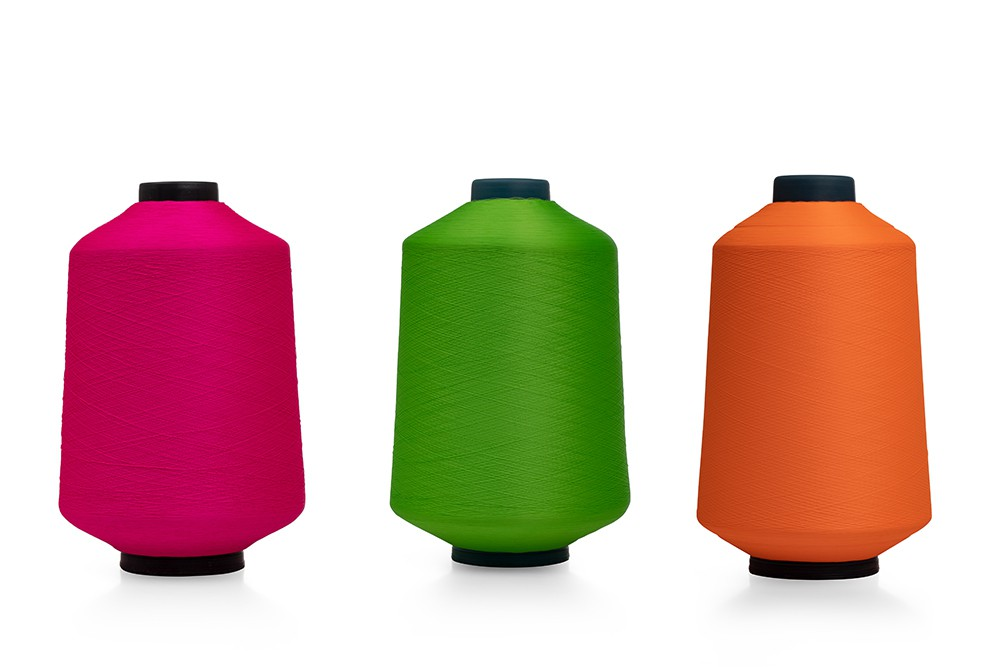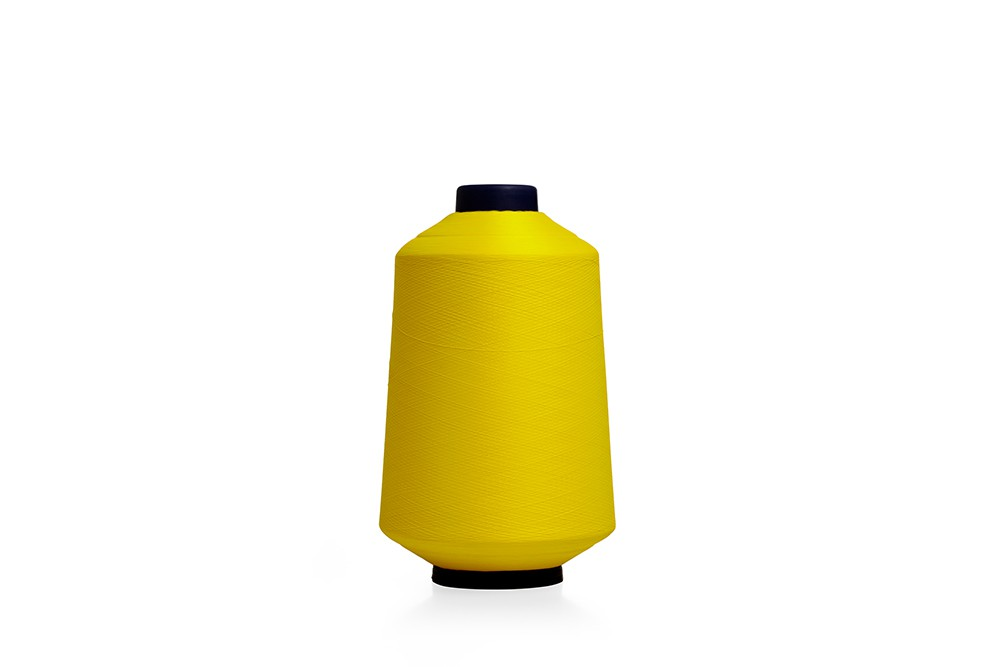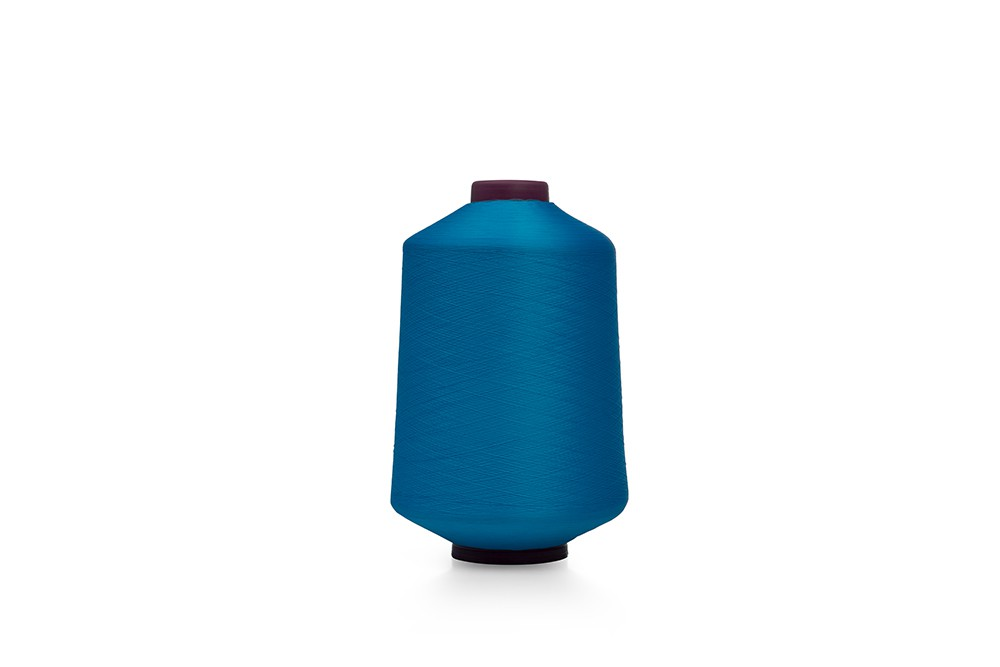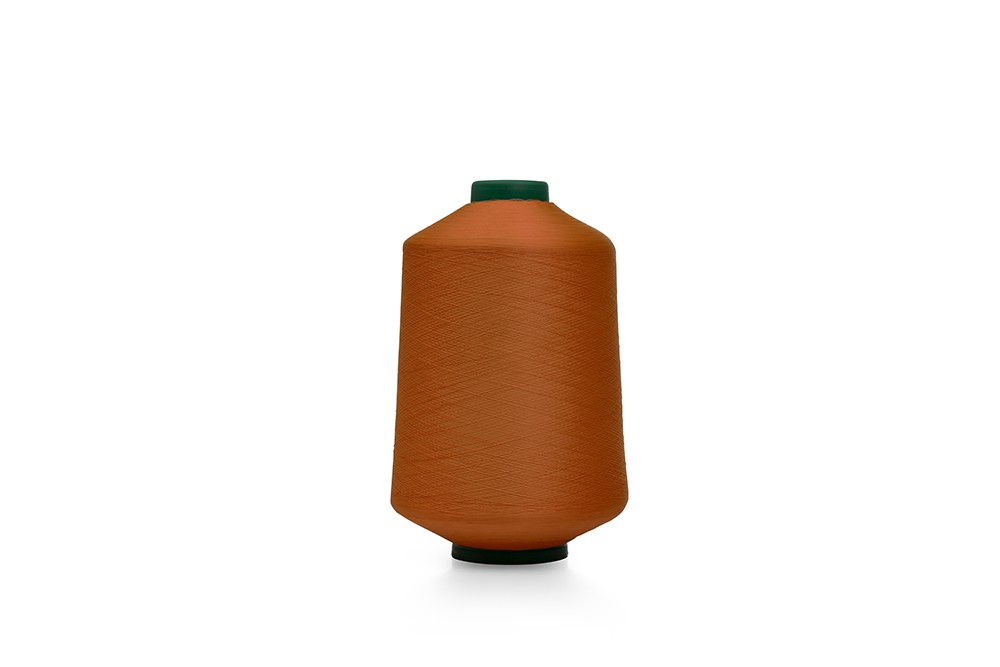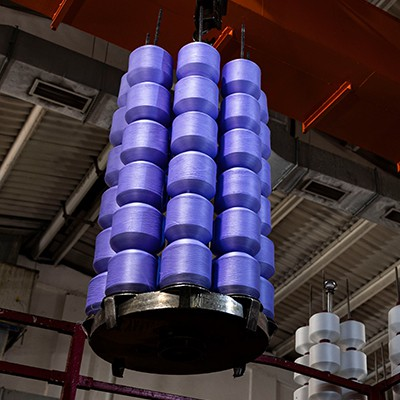## Dyed Nylon Yarn

WHAT IS NYLON YARN?

Polyamide, which is a synthetic fiber, also known as nylon yarn among the people, is the first synthetic fiber produced in the world. It is obtained from hard coal and petroleum products, and classified as Synthetic fiber. Two types of yarn, nylon and perlon, are produced from Polyamide fiber.

Denier Dtex Filament Quality Matteness Section Warping Direction
Shiny Semi-Matte Full Matte
35 38 24 N6.0 X X X ROUND S/Z (PARALLEL)
50 55 36 N6.0 X X X ROUND S/Z (PARALLEL)
50 55 48 N6.0 X X X ROUND S/Z (PARALLEL)
68 75 36 N6.0 X X X ROUND S/Z (PARALLEL)
75 83 36 N6.0 X X X ROUND S/Z (PARALLEL)
75 83 48 N6.0 X X X ROUND S/Z (PARALLEL)
100 111 36 N6.0 X X X ROUND S/Z (PARALLEL)
100 111 48 N6.0 X X X ROUND S/Z (PARALLEL)
135 150 48 N6.0 X X X ROUND S/Z (PARALLEL)
150 167 36 N6.0 X X X ROUND S/Z (PARALLEL)
150 167 48 N6.0 X X X ROUND S/Z (PARALLEL)
150 167 72 N6.0 X X X ROUND S/Z (PARALLEL)
150 167 96 N6.0 X X X ROUND S/Z (PARALLEL)
170 188 36 N6.0 X X X ROUND S/Z (PARALLEL)
200 222 72 N6.0 X X X ROUND S/Z (PARALLEL)
200 222 96 N6.0 X X X ROUND S/Z (PARALLEL)
300 335 72 N6.0 X X X ROUND S/Z (PARALLEL)
300 335 96 N6.0 X X X ROUND S/Z (PARALLEL)